ISEE Middle Level Math : How to find the square root

Example Questions

Example Question #261 : How To Find The Square Root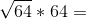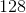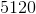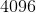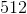Explanation:

Simplify by taking the square root before performing the multiplication: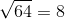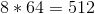Answer:Example Question #262 : How To Find The Square Root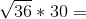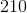Explanation:

First take care of the radical: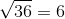Then take the product: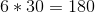Answer:Example Question #263 : How To Find The Square Root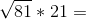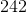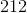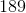Explanation:

First find the square root: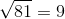Then find the product: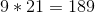Answer:Example Question #264 : How To Find The Square Root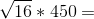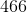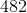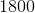Explanation:

First find the square root: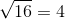Then multiply: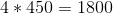Answer:Example Question #265 : How To Find The Square Root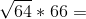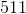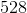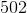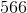Explanation:

First find the square root:Next, find the product: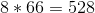Answer:Example Question #266 : How To Find The Square Root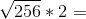Explanation:

First find the square root: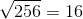Then multiply: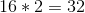Answer:Example Question #267 : How To Find The Square Root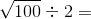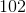Explanation:

First find the square root: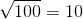Then divide: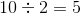Answer:Example Question #268 : How To Find The Square Root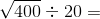Explanation:

First find the square root: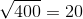Then divide: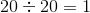Answer:Example Question #269 : How To Find The Square Root

Solve: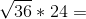Explanation:

First find the square root:Then multiply: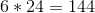Answer:Example Question #270 : How To Find The Square Root

Solve: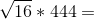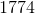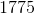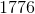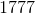First find the square root:Then multiply: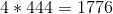Answer: